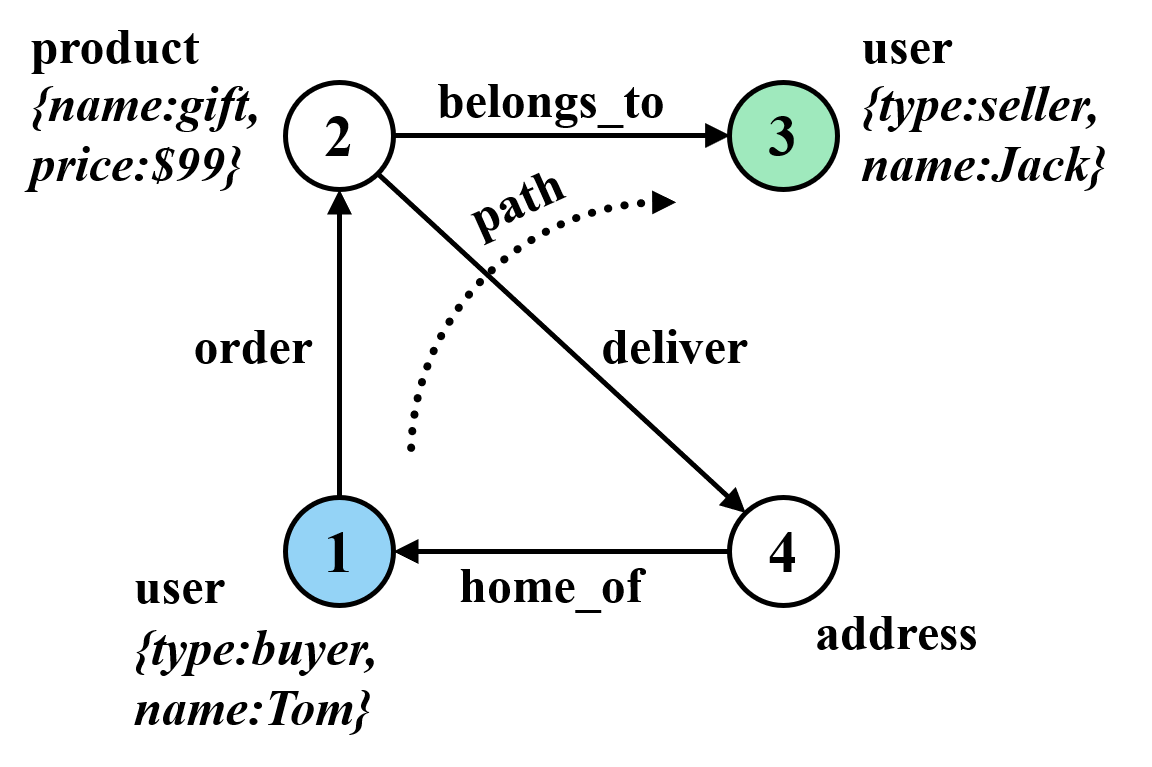# 图交互式分析引擎

GraphScope的交互查询引擎（简称GIE）是一个分布式系统，它为不同经验的用户提供了一个易用的交互式环境，支持海量复杂图数据上的 实时分析与交互探索 。该引擎支持 Gremlin 语言表达的交互图查询，并提供了自动化和用户透明的分布式并行执行。

## Apache TinkerPop

Apache TinkerPop 是基于Gremlin语言开发交互式图应用的一个开源框架和事实标准。GIE通过TinkerPop提供的 Gremlin Server 接口，实现了与TinkerPop生态无缝集成，从而用户可以直接采用诸如 Gremlin Console 的开发工具或通过Java和Python等多种语言接口编写应用逻辑。

## 利用Python（Gremlin）连接GraphScope

```import graphscope
from graphscope.dataset import load_ldbc

# 创建一个新的交互会话，载入LDBC示例图数据
# 随后返回一个Gremlin查询提交入口
sess = graphscope.session(num_workers=2)
graph = load_ldbc(sess, prefix='/path/to/ldbc_sample')
interactive = sess.gremlin(graph)

# 下面两句Gremlin示例查询分别计算图中顶点和边的总数
node_num = interactive.execute('g.V().count()').one()
edge_num = interactive.execute("g.E().count()").one()
```

```print(interactive.graph_url)
```

```ws://your-endpoint:your-ip/gremlin
```

## 利用Java（Gremlin）连接GraphScope

TinkerPop同时支持Java语言按类似方式访问，详见Gremlin-Java的 官方文档

## Gremlin Console（开发控制台）

Gremlin Console 为开发者提供了一个与GraphScope存储的图数据进行交互的控制台，也叫做REPL环境（read-evaluate-print loop）。下面描述如何利用上文获得的URL，安装和配置Gremlin Console以连接GraphScope的步骤：

1.安装Gremlin Console依赖的Java运行时环境，版本需要满足[8, 12)。

2.从 Apache TinkerPop 下载适当版本的Gremlin Console。

```wget https://archive.apache.org/dist/tinkerpop/3.4.8/apache-tinkerpop-gremlin-console-3.4.8-bin.zip
```

3.解压缩下载的文件。

```unzip apache-tinkerpop-gremlin-console-3.4.8-bin.zip
```

4.进入解压缩的目录。

```cd apache-tinkerpop-gremlin-console-3.4.8
```

5.在 conf 子目录创建一个名为 graphscope-remote.yaml 的文本文件以配置URL。具体内容如下所示，其中的 your-endpointyour-port 需要分别替换为从GraphScope会话得到的URL中对应的主机名（或IP）和端口。

```hosts: [your-endpoint]
port: your-port
serializer: { className: org.apache.tinkerpop.gremlin.driver.ser.GryoMessageSerializerV1d0, config: { serializeResultToString: true }}
```

6.输入下列命令启动Gremlin Console。

```bin/gremlin.sh
```

7.在 gremlin> 提示符下，输入下列命令连接到对应的GraphScope会话；第二条命令切换到远程模式，从而接下来输入的所有Gremlin查询都被自动传输到（远程）GraphScope执行。

```:remote connect tinkerpop.server conf/graphscope-remote.yaml
:remote console
```

8.现在你可以尝试一些简单的Gremlin查询了！例如 `g.V().limit(1)` 。当你完成交互，输入下列命令可以退出Gremlin Console。

```:exit
```

## Gremlin编程入门–101

GIE以忠实保留Gremlin编程模型为设计目标，从而让已有的应用只需最小化的修改就可以扩展到大规模计算集群。在此我们提供一个Gremlin的总体介绍，特别是其中包含的图数据模型和查询语言等关键概念。更详细和完整的介绍，请参考 TinkerPop reference

### 图数据模型

Gremlin允许用户在属性图模型上定义特设（ad-hoc）遍历查询。一个属性图是一个有向图，其中的顶点和边可以拥有一组属性。图中的每个对象（点或边）都有一个唯一标识（`ID`）和一个类别名称（`label`）指定其类型或角色。每个属性是一个包含属性名和属性值的（键-值）对，其所属对象的 `ID` 加上属性名可以唯一确定属性值。### 查询语言

Gremlin语言丰富灵活的表达能力主要来自于它对 嵌套遍历 的支持，它允许一个（子）查询或遍历被包含在另一个操作中，作为一个可调用的函数被包裹操作用于处理其每一个输入。函数的声明和作用都由包裹操作的语义决定。

### 一个例子

```g.V('account').has('id','2').as('s')
.out('k-1..k', 'transfer')
.with('PATH_OPT', 'SIMPLE')
.endV()
.where(out('transfer').eq('s'))
.path().limit(1)
```

## Gremlin兼容性（对比TinkerPop）

GIE支持Apache TinkerPop定义的属性图模型和Gremlin遍历查询，且实现了一个与TinkerPop 3.3和3.4版本兼容的 Websockets 服务接口。除此之外，我们扩展了一些语法糖来进一步引入一些简洁明了的expression表示。下面我们列出当前实现和Apache TinkerPop规范的主要差一点（其中一些差异会有机会消除、另一些是目前GraphScope定位的场景差异造成的不同设计选择）。

### 属性图模型约束

• Schema（模式）约束：每个图的数据需要满足事先定义的Schema，包括点、边的类型名称（label）和属性名及值类型。

• 主键约束：每个顶点类型需要包含一个用户可自定义的主键（属性），同时系统会为每个点和边对象，自动分配产生一个字符串类型的唯一标识（ID）。对于点来说，ID编码了类型（label）和用户自定义主键信息。

• 每个点或边的属性，可以包含下列类型的属性值：`int``long``float``double``String``List<int>``List<long>``List<String>`

### 尚不支持的功能特性

• 图修改操作。

• Lambda和Groovy表达式或自定义函数，例如：`.map{<expression>}``.by{<expression>}``.filter{<expression>}` 函数，`1+1``System.currentTimeMillis()` 等表达式或Java调用等等。

• 定制Gremlin图遍历策略（traversal strategies），即查询优化由GraphScope系统自动完成。

• 事务。

• 二级索引目前尚未支持（用户定义的主键会被自动索引）。

### 支持的Gremlin操作

• Source（输入图），如：

```//V
g.V()
g.V(id1, id2)

//E
g.E()
```
• Filter（过滤），如：

```//hasLabel
g.V().hasLabel("labelName")
g.V().hasLabel("labelName1", "labelName2")

//has
g.V().has("attrName")
g.V().has("attrName", attrValue)
g.V().has("labelName", "attrName", attrValue)
g.V().has("attrName", eq(1))
g.V().has("attrName", neq(1))
g.V().has("attrName", lt(1))
g.V().has("attrName", lte(1))
g.V().has("attrName", gt(1))
g.V().has("attrName", gte(1))
g.V().has("attrName", within([1,2,3]))
g.V().has("attrName", without([1,2,3]))
g.V().has("attrName", inside(10, 20))
g.V().has("attrName", outside(10, 20))

// P.not
g.V().has("attrName", P.not(eq(10)))

//is
g.V().values("age").is(gt(70))

//通过expression实现过滤 (`expr()` 语法糖)
g.V().where(expr('@.age > 20')) //@.age 代表head节点的age属性
g.V().as('a').out().as('b').where(expr('@a.age <= @b.age'))  //@a.age 代表 "a" 节点的age属性
g.V().where(expr('30 within @.a'))  //head节点的a属性是整数数组类型
//project with expression (`expr()` 语法糖)

//通过expression实现位运算
g.V().select(expr("@.number & 2")) //head节点的number属性是整型
g.V().select(expr("@.number | 2"))
g.V().select(expr("@.number ^ 2"))
g.V().select(expr("@.number << 2"))
g.V().select(expr("@.number >> 2"))
g.V().where(expr("@.number & 64 != 0"))

//通过expression实现算数运算
g.V().select(expr("@.number + 2"))
g.V().select(expr("@.number - 2"))
g.V().select(expr("@.number * 2"))
g.V().select(expr("@.number / 2"))
g.V().select(expr("(@.number + 2) / 4 + (@.age * 10)")) //head节点的number和age属性都是整型

//通过expression实现指数运算
g.V().select(expr("@.number ^^ 3"))
g.V().select(expr("@.number ^^ -3"))

//where
g.V().where(out().count().is(gt(4)))

//dedup
g.V().out().dedup()
g.V().out().dedup().by("name")
g.V().as("a").out().dedup("a")
g.V().as("a").out().dedup("a").by("name")

//range
g.V().out().limit(100)
g.V().out().range(10, 20)

//TextP.*
g.V().has("attrName", TextP.containing("substr"))
g.V().has("attrName", TextP.notContaining("substr"))
g.V().has("attrName", TextP.startingWith("substr"))
g.V().has("attrName", TextP.notStartingWith("substr"))
g.V().has("attrName", TextP.endingWith("substr"))
g.V().has("attrName", TextP.notEndingWith("substr"))
```
• Map（映射），如：

```//constant
g.V().out().contant(1)
g.V().out().constant("aaa")

//id
g.V().id()

//label
g.V().label()

//otherV
g.V().bothE().otherV()

//as...select
g.V().as("a").out().out().select("a")
g.V().as("a").out().as("b").out().as('c').select("a", "b", "c")
```
• FlatMap（多重映射），如：

```//out/in/both
g.V().out()
g.V().in('knows')

//outE/inE/inV/outV
g.V().outE('knows').inV()
g.V().inE().bothV()

//path expansion (语法糖)
//找到所有从 `V()` 开始通过 `knows` 边类型向外扩展[2, 4)跳的所有简单路径（点不重复），并且只保存path的最末端点
g.V().out('2..4', 'knows').with('PATH_OPT', 'SIMPLE').with('RESULT_OPT', 'END_V').endV()
//找到所有从 `V()` 开始通过 `knows` 边类型向外扩展[2, 4)跳的所有任意路径（点可重复），并且只保存path的最末端点
g.V().out('2..4', 'knows').with('PATH_OPT', 'ARBITRARY').with('RESULT_OPT', 'ALL_V')

//properties
g.V().values("name")
g.V().valueMap() // 输出所有属性
g.V().valueMap("name")
g.V().valueMap("name", "age")
```
• Aggregate（聚合），如：

```//global count
g.V().out().count()
g.V().where(out().in().count().is(0))

//fold
g.V().fold()
g.V().values("name").fold()

//groupCount
g.V().out().groupCount()
g.V().values("name").groupCount()

//groupBy
g.V().out().group()
g.V().out().group().by("name")
g.V().out().group().by().by("name")

//groupBy多个keys，并且为每个key设置别名
g.V().group().by(values("name").as("name"), values("age").as("age"))
//groupBy多个values，并且为每个value设置别名
g.V().group().by().by(min().as("min"), max().as("max"))

//global max/min
g.V().values("age").max()
g.V().values("age").min()

//global sum
g.V().values("age").sum()
```
• Loop（循环），如：

```g.V().match(
__.as('a').out().as('b'),
__.as('b').out().as('c')
).select('a', 'c')
```
• Limit（top K，即取前k个结果）。

### 已知限制

GraphScope暂时不支持下列Gremlin操作（会逐步支持）：

• Repeat (可以通过path expansion语法糖实现)

• path()/simplePath() (可以通过path expansion语法糖实现)

• Local (基于集合的local计算，i.e. count(local), dedup(local), …)

• Branch

• Explain（查询计划解释）

• Profile（查询执行性能分析）

• Sack（自定义状态计算）

• Subgraph（计算子图，目前实现了一个简化版本，支持抽取子图写入Vineyard存储）

• Cap（访问自定义状态）

• `GraphComputer` 接口（例如PageRank和ShortestPath）；这部分功能GraphScope通过图分析引擎和NetworkX兼容接口提供。